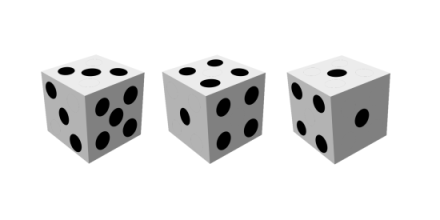# Lake Wobegon Dice

Garrison Keillor’s fictional Lake Wobegon is a place “where all the children are above average.” Donald Knuth alluded to this in his exercise regarding “Lake Wobegon Dice,” a set of dice where the roll of each die is (probably) above average.

Let A be a six-sided die with a 5 on one side and 3’s on the other sides.

Let B and C be six-sided dice with 1’s on two sides and 4’s on four sides.Then the probabilities

Pr( A > (A + B + C)/3 )
Pr( B > (A + B + C)/3 )
Pr( C > (A + B + C)/3 )

are all greater than 1/2.

To see this, list out all the possible rolls where each die is above average. The triples show the value of A, B, and C in that order. We have A above average if the rolls are

(3, 1, 1)
(3, 1, 4)
(3, 4, 1)
(5, *, *)

These outcomes have probability 5/54, 10/54, 10/54, and 1/6. The total is 34/54 = 17/27 > 1/2.

We have B above average when the rolls are

(3, 4, *)
(5, 4, 1)

which have probability 5/9 and 1/27 for a total of 16/27 > 1/2. And C is the same as B.

It seems paradoxical for three different dice to each have a probability better than 1/2 of being above average. The resolution is that the three events

A > (A + B + C)/3
B > (A + B + C)/3
C > (A + B + C)/3

are not exclusive. For example, in the roll (3, 4, 1) both A and B are above the average of 8/3.

I ran across this in The Art of Computer Programming, Volume 4, Pre-fascicle 5A, Exercise 3. Fascicle 5 has since been published, but I don’t have a copy. I don’t know whether this exercise made it into the published version. If it did, the page number may have changed.

## One thought on “Lake Wobegon Dice”

1. Jakub Narębski

Is it different take on non-transitive dice (dice A wins on average with B, B wins with C, C wins with A)?